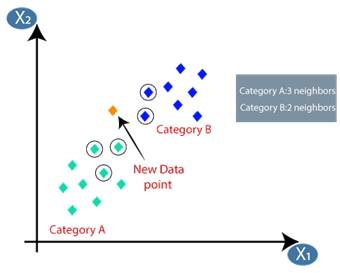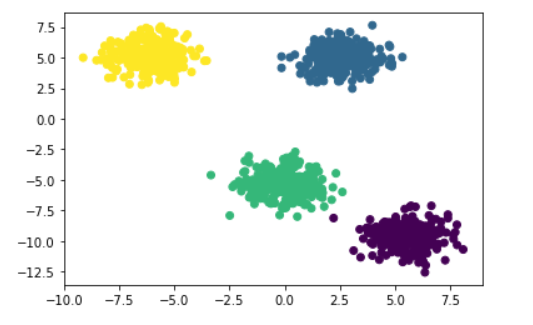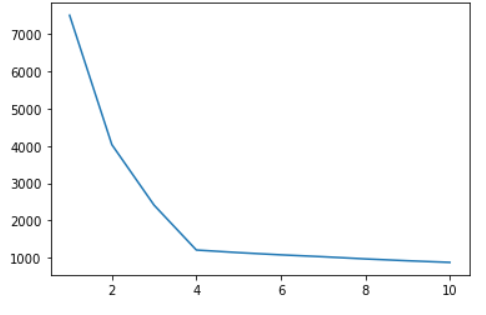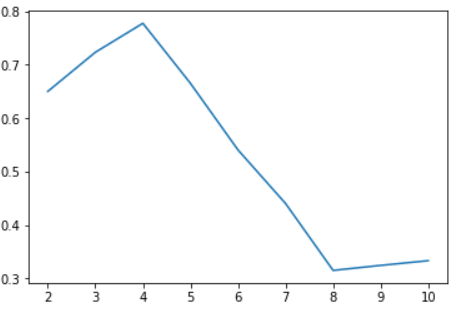# Determining the Optimal K for K-Means Algorithm

## Introduction

K Nearest Neighbors (KNN) is one of the simplest algorithms in Machine Learning. It is a supervised algorithm and is used for classification and regression problems.  It works on the intuition that similar things lie close to each other, i.e., things with similar features would lie close to each other.

Let us understand the working of the algorithm with the help of a simple example.

Let us suppose that we are a clothing company and have a classification problem. We have to predict the size of the T-shirt that would fit a person based on only two features(for simplicity), i.e., the height of the person and the person's weight. We already have a dataset of our customers with their heights and weights and the T-shirt size they bought. Let us again assume for simplicity that there are only two T-shirt sizes, i.e., category A which represents the size Medium, and category B, which represents the size Large. Now there is a customer whose height and weight is known, and we want to suggest to them the correct size of T-shirt they should buy (New data point).Source: javatpoint.com

We plot our customers' data, and let's say we run the knn algorithm for the new data point for K=5, i.e., the algorithm will identify five nearest neighbors (in terms of distance) for the new data point. We found out that 3 of the nearest neighbors are from category A and 2 of them are from category B. Hence, our KNN algorithm would predict the output class of the new data point to be Category A, i.e., Medium size T-Shirt.

Choosing the Right K Value

Choosing the right K value is important for getting accurate results with the algorithm. Two cases could arise:-

1.  The K value is too less, and it leads to underfitting if the K value is too less, i.e., if we select K=1 or K=2, we would be only looking at a very small number of neighbors and hence won't be utilizing the full scope of the data.

2.  The K value is too large, which may lead to overfitting. If the K value is too large, we might consider a lot of outliers, which would lead to inaccurate results.

There are two methods we will be studying that helps us to determine the optimal k value:-

1. Elbow Method
2. Silhouette Method

We will be creating an artificial dataset using the make_blobs library in sklearn. We will be making 4 clusters, and we will verify that both the methods give us the optimal value of K, i.e., 4. We will be having 1000 data points and two features so that it is to plot the data.

Now we will plot the data and see how it looks.## Elbow Method

In the elbow method, we run the K-Means algorithms on different k values and calculate the Within Cluster Sum of Squared Errors(WSS) for these values of K.

WSS is the sum of the square of the distance of each point from its center in the cluster(sum of squared errors), and this distance can be calculated with any standard distance metric such as the Euclidean Distance.

If we plot WSS vs. K, the K plot at which the WSS starts to decrease abruptly(this point looks like an elbow, hence the name of the method) followed by a gradual decrease is the optimal K value.

Let's implement this method in code and see its results on our data.

We obtain the below K vs. Wss plot.As you can see, there is an elbow formation at K=4. Hence, we have verified that K=4 is our optimal value with this method.

We might not have a clear elbow for every kind of data. In such cases, we use another method called the Silhouette Method.

## The Silhouette Method

The silhouette value measures how similar a point is to its cluster (cohesion) compared to other clusters (separation).

The silhouette score lies between the range of -1 and 1. A higher silhouette score indicates that the point is placed in the correct cluster.

If we plot  K vs. the Silhouette score, the point at which the silhouette reaches its maximum is the optimal value of K.

We can easily calculate the Silhouette score in python using the sklearn library.

If we plot K vs. silhouette score, we get the below plot.We get the maximum score at 4; hence it is the optimal K value.

1. When should we not use the Elbow Method?
As the elbow method only takes the Euclidean distance into account, there would be cases in which there is no clear "elbow" point obtained on plotting the WSS values for each K value. In such cases, we should not use the elbow method and resort to the silhouette method

2. Why do we not check the silhouette score for K=1?
The Silhouette score measures a point’s similarity to its own cluster with respect to another cluster; it does not make sense to check for K=1.
Also, the silhouette score for a cluster containing a single point is zero.

3. What is the make_blobs library in sklearn?
It is a library used to generate test data sets for clustering, just like we did in this blog. It generates isotropic Gaussian blobs for clustering.

## Key Takeaways

We learn about the K-means algorithm and the importance of choosing the right K value for the algorithm. We explored the Elbow Method and the Silhouette Method, which helped us determine the optimal K value for our dataset. We also implemented these two methods in python on a random clustered dataset.